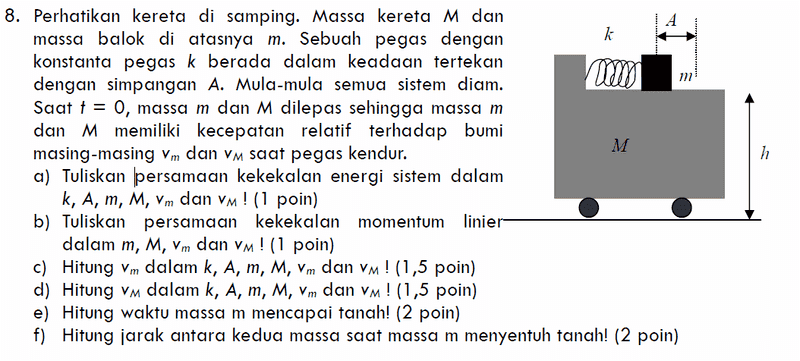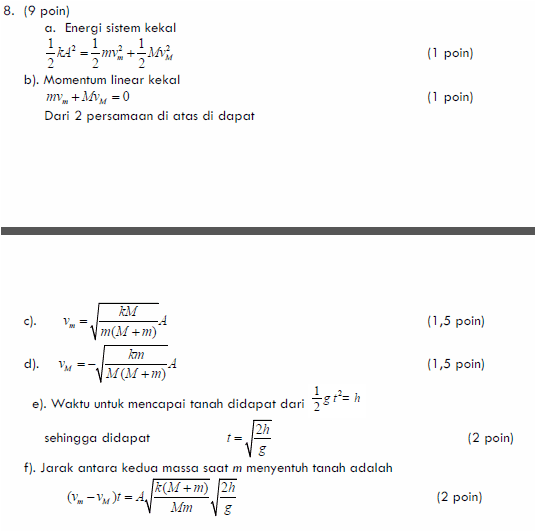# Is the momentum conserved ?

terryds

## Homework Statement

http://www.sumoware.com/images/temp/xzqmibbrldrafpgj.png [Broken]

At first, the system is statics. Then, a spring with spring constant k is pressed with Δx = A. At t=0, mass m and M are released so mass m and M have relative velocity to the Earth (vm and vM)
Determine both velocity in k, A, m, M

E = E'
p = p'

## The Attempt at a Solution

Actually, I've got the solution in my book.
The solution is to use the conservation of energy and momentum.

But, what I don't understand is why using conservation of momentum ?
I think the momentum is not conserved, since there is force of the spring that makes both vm and vM goes in the same direction.
I imagine it. First, the system is static (initial momentum is zero). Then, the object m and M is moving in the same direction (so, I think that the final momentum is not zero)

Last edited by a moderator:

I think the momentum is not conserved, since there is force of the spring that makes both vm and vM goes in the same direction.
The spring exerts two forces. The left end of the spring pushes mass M to the left. The right end of the spring pushes mass m to the right.

I imagine it. First, the system is static (initial momentum is zero). Then, the object m and M is moving in the same direction (so, I think that the final momentum is not zero)

Suppose mass m is not firmly attached to the spring. Then m is a projectile. Shooting a projectile creates recoil. The gun and the bullet move in opposite directions.

•terryds
I think the momentum is not conserved, since there is force of the spring that makes both vm and vM goes in the same direction.
That is where you are wrong. They move in opposite directions. Since everything is static when t<0, the total momentum is 0. Therefore, at t=0+, $m\cdot v_{m}+M\cdot v_{M}=0$.
Energy budget: All relevant energy is stored in the spring as $k\cdot m\cdot A$
After a while, m has traveled A and the spring is no longer pushing m and there is no energy left in the spring. Since there is no mention of friction, all energy is conserved. This means that all energy is converted to kinetic energy: $\frac{1}{2}m\cdot v_{m}^{2}+\frac{1}{2}M\cdot v_{M}^{2}$
Now m starts pulling at the spring, which again pulls at M....

•terryds
Homework Helper
Gold Member
Is this the exact wording of the question?
m and M are released so mass m and M have relative velocity to the Earth
Yes, but not instantly nonzero.
Determine both velocity in k, A, m, M
...as functions of t, or maybe of x? Or does it perhaps ask for the maximum velocities?

terryds
Is this the exact wording of the question?

Yes, but not instantly nonzero.

...as functions of t, or maybe of x? Or does it perhaps ask for the maximum velocities?

No, it's not the exact wording of the question. Actually, the question is written in Bahasa Indonesia. Sorry for my bad translate..It asks the velocity in as function of A or we may say Δx

But, I've got the solution below

Conservation of Energy
0.5kA^2 = 0.5mvm2 + 0.5MvM2

Conservation of Linear Momentum
mvm + MvM = 0

From 2 equations above, we can solve for velocities
vm = √( (kM)/(m(M+m) ) * A
vM = - √( (km)/(M(M+m) ) * A

If you see anything wrong with the solution above, please tell me..
Thanks

Homework Helper
Gold Member
It asks the velocity in as function of A or we may say Δx
A and Δx are not the same thing. A is a constant, the value of Δx at time t=0. Δx is a variable over time.
Expressing the velocities at time t as a function of Δx (at time t) and A makes sense.
Conservation of Energy
0.5kA^2 = 0.5mvm2 + 0.5MvM2
No, the energy on the left of that equation is the initial energy, which will equal the maximum KE. But at some arbitrary time t, the total energy will involve both KE (with some lower velocities) and some PE in the spring (with less extension than A).
Looking through the list of questions in Indonesian, none of them finish off by listing "k, A, m, M". Which question is it?

terryds
A and Δx are not the same thing. A is a constant, the value of Δx at time t=0. Δx is a variable over time.
Expressing the velocities at time t as a function of Δx (at time t) and A makes sense.

No, the energy on the left of that equation is the initial energy, which will equal the maximum KE. But at some arbitrary time t, the total energy will involve both KE (with some lower velocities) and some PE in the spring (with less extension than A).
Looking through the list of questions in Indonesian, none of them finish off by listing "k, A, m, M". Which question is it?

It asks for velocity as a function of A
Take a look at question c and d in the Indonesian question

I think the final elastic potential energy is zero since Δx is zero at the last position,, right ?

Homework Helper
Gold Member
It asks for velocity as a function of A
Take a look at question c and d in the Indonesian question
Yes, I looked at those, but c (for example) seems to say: "express vm in terms of k, A, M, m, vm and vM", which makes no sense.
I think the final elastic potential energy is zero since Δx is zero at the last position,, right ?
There is no final state. It will oscillate forever (there being no friction). The elastic PE will decline to zero, yes, and at that point the velocities will be as you calculated, but the masses will continue to move apart, the velocities decreasing to zero and the elastic PE returning to its original value (but now as extension instead of compression). Then it will all go into reverse, and continue ad tedium.
I suggest that c and d are written wrongly. E.g. c should say "hitung vm dalan k, A, M, m dan Δx".

Mentor
Apparently the small mass m is not fixed to the spring. It is launched from the cart.

I found a copy of the problem on a web site and had Google Translate do its thing here.
It's the final problem on the page.

terryds
Yes, I looked at those, but c (for example) seems to say: "express vm in terms of k, A, M, m, vm and vM", which makes no sense.

There is no final state. It will oscillate forever (there being no friction). The elastic PE will decline to zero, yes, and at that point the velocities will be as you calculated, but the masses will continue to move apart, the velocities decreasing to zero and the elastic PE returning to its original value (but now as extension instead of compression). Then it will all go into reverse, and continue ad tedium.
I suggest that c and d are written wrongly. E.g. c should say "hitung vm dalan k, A, M, m dan Δx".

So,
0.5 k Δx^2 = 0.5mvm2 + 0.5mvM2 + 0.5 k Δx' ^ 2
Then,
mvm + MvM = 0
Then, we can solve it using two equations above.

And, I think the question to the answer
vm = √( (kM)/(m(M+m) ) * A
Should the question be "The velocity when the spring reached the equilibrium position ?"
I know that it will oscillate forever, but the velocity when the spring position in equilibrium ( at a very small time, because it will continue oscillating)

Homework Helper
Gold Member
Should the question be "The velocity when the spring reached the equilibrium position ?"
Possibly. Is vm = √( (kM)/(m(M+m) ) * A the answer in the book?

terryds
Apparently the small mass m is not fixed to the spring. It is launched from the cart.

I found a copy of the problem on a web site and had Google Translate do its thing here.
It's the final problem on the page.

Thanks a lot
Possibly. Is vm = √( (kM)/(m(M+m) ) * A the answer in the book?
This is the answer in my bookSo, the question is the velocity when the spring reached the equilibrium position, right ?
Hmm.. It seems I missed translating the last part of the question.. Sorry for my mistake
The 'Saat t = 0, massa m dan M dilepas sehingga massa m dan M memiliki kecepatan relatif terhadap bumi masing-masing vm dan vM saat pegas kendur.'
means ' at t=0, mass m and M are released so mass m and M have relative velocity to the earth vm and vM when the spring is not in extension nor compression (at the equilibrium position)'

•